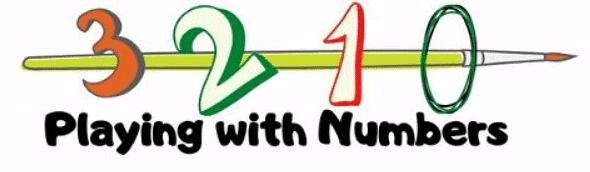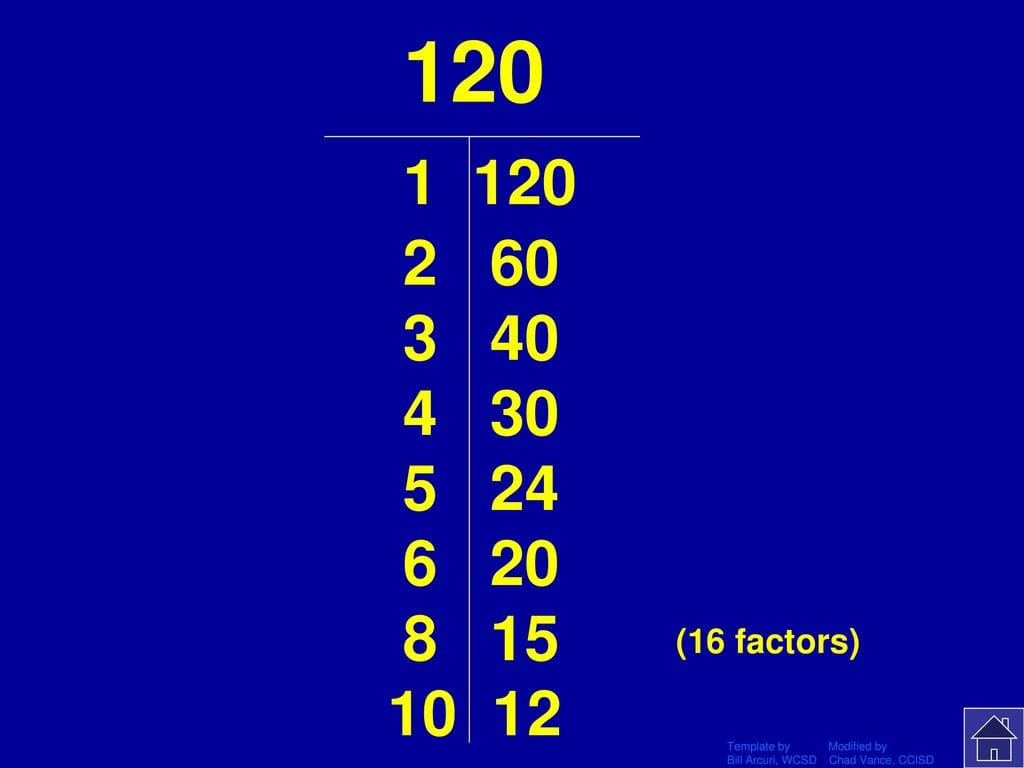Worksheet Questions: Playing with Numbers- 1

# Worksheet Questions: Playing with Numbers- 1 - Notes | Study Mathematics (Maths) Class 6 - Class 6

 1 Crore+ students have signed up on EduRev. Have you?Ques 1: State whether the following statements are True or False:
(a) The sum of three odd numbers is even.
Ans: False 3 + 5 + 7 = 15, i.e., odd

(b) The sum of two odd numbers and one even number is even.
Ans: True 3 + 5 + 6 = 14, i.e., even

(c) The product of three odd numbers is odd.
Ans: True 3 x 5 x 7 = 105, i.e., odd

(d) If an even number is divided by 2, the quotient is always odd.
Ans: False 4 ÷ 2 = 2, i.e., even

(e) All prime numbers are odd.
Ans: False 2 is a prime number and it is also even

(f) Prime numbers do not have any factors.
Ans: False 1 and the number itself are factors of the number

(g) Sum of two prime numbers is always even.
Ans: False 2 + 3 = 5, i.e., odd

(h) 2 is the only even prime number.
Ans: True

(i) All even numbers are composite numbers.
Ans: False 2 is a prime number

(j) The product of two even numbers is always even.
Ans: True 2 x 4 = 8, i.e., even

Ques 2: Find all the multiple of 13 up to 100.
Ans: 13, 26, 39, 52, 65, 78, 91

Ques 3: Write all the factors of 120.
Ans: 1, 2, 3, 4, 5, 6, 8, 10, 12, 15, 20, 24, 30, 40, 60, 120Factors of 120

Ques 4: Circle the numbers below which are multiples of 45.
Ans: 270, 295, 305, 315, 333, 360, 400

Ques 5: The numbers 13 and 31 are prime numbers. Both these numbers have same digits 1 and 3. Find such pairs of prime numbers up to 100.
Ans: 17, 71
37, 73
79, 97

Ques 6: Write down separately the prime and composite numbers less than 20.
Ans: Prime numbers less than 20 are
2, 3, 5, 7, 11, 13, 17, 19
Composite numbers less than 20 are
4, 6, 8, 9, 10, 12, 14, 15, 16, 18

Ques 7: What is the greatest prime number between 1 and 10?
Ans: Prime numbers between 1 and 10 are 2, 3, 5, and 7. Among these numbers, 7 is the greatest.

Ques 8: Express the following as the sum of two odd primes.
(a) 44
(b) 36
(c) 24
(d) 18
Ans: (a) 44 = 37 + 7
(b) 36 = 31 + 5
(c) 24 = 19 + 5
(d) 18 = 11 + 7

Ques 9: Write seven consecutive composite numbers less than 100 so that there is no prime number between them.
Ans: Between 89 and 97, both of which are prime numbers, there are 7 composite numbers. They are
90, 91, 92, 93, 94, 95, 96
Numbers Factors
90 1, 2, 3, 5, 6, 9, 10, 15, 18, 30, 45, 90
91 1, 7, 13, 91
92 1, 2, 4, 23, 46, 92
93 1, 3, 31, 93
94 1, 2, 47, 94
95 1, 5, 19, 95
96 1, 2, 3, 4, 6, 8, 12, 16, 24, 32, 48, 96

Ques 10: Write a digit in the blank space of each of the following numbers so that the number formed is divisible by 11:
(a) 92 ___ 389
(b) 8 ___9484
Ans:
(a) 92_389
Let a be placed in the blank.
Sum of the digits at odd places = 9 + 3 + 2 = 14
Sum of the digits at even places = 8 + + 9 = 17 + a
Difference = 17 + a − 14 = 3 + a
For a number to be divisible by 11, this difference should be zero or a multiple of 11.
If 3 + a = 0, then
a = − 3
However, it cannot be negative.
A closest multiple of 11, which is near to 3, has to be taken. It is 11itself.
3 + a = 11
a = 8
Therefore, the required digit is 8.
(b) 8_9484
Let a be placed in the blank.
Sum of the digits at odd places = 4 + 4 + a = 8 + a
Sum of the digits at even places = 8 + 9 + 8 = 25
Difference = 25 − (8 + a)
= 17 − a
For a number to be divisible by 11, this difference should be zero or a multiple of 11.
If 17 − a = 0, then
a = 17
This is not possible.
A multiple of 11 has to be taken. Taking 11, we obtain
17 − a = 11
a = 6
Therefore, the required digit is 6.

Ques 11: A number is divisible by both 5 and 12. By which other number will that number be always divisible?
Ans: Factors of 5 = 1, 5
Factors of 12 = 1, 2, 3, 4, 6, 12
As the common factor of these numbers is 1, the given two numbers are co- prime and the number will also be divisible by their product, i.e. 60, and the factors of 60, i.e., 1, 2, 3, 4, 5, 6, 10, 12, 15, 20, 30, 60.

Ques 12: A number is divisible by 12. By what other number will that number be divisible?
Ans: Since the number is divisible by 12, it will also be divisible by its factors i.e., 1, 2, 3, 4, 6, 12. Clearly, 1, 2, 3, 4, and 6 are numbers other than 12 by which this number is also divisible.

Ques 13: The product of three consecutive numbers is always divisible by 6. Verify this statement with the help of some examples.
Ans: 2 * 3 * 4 = 24, which is divisible by 6
9 * 10 * 11 = 990, which is divisible by 6
20 * 21 * 22 = 9240, which is divisible by 6

Ques 14: The sum of two consecutive odd numbers is divisible by 4. Verify this statement with the help of some examples.
Ans: 3 + 5 = 8, which is divisible by 4
15 + 17 = 32, which is divisible by 4
19 + 21 = 40, which is divisible by 4

Ques 15: Determine if 25110 is divisible by 45.
[Hint: 5 and 9 are co-prime numbers. Test the divisibility of the number by 5 and 9].
Ans: 45 = 5 * 9
Factors of 5 = 1, 5
Factors of 9 = 1, 3, 9
Therefore, 5 and 9 are co-prime numbers.
Since the last digit of 25110 is 0, it is divisible by 5.
Sum of the digits of 25110 = 2 + 5 + 1 + 1 + 0 = 9
As the sum of the digits of 25110 is divisible by 9, therefore, 25110 is divisible by 9.
Since the number is divisible by 5 and 9 both, it is divisible by 45.

The document Worksheet Questions: Playing with Numbers- 1 - Notes | Study Mathematics (Maths) Class 6 - Class 6 is a part of the Class 6 Course Mathematics (Maths) Class 6.
All you need of Class 6 at this link: Class 6

## Mathematics (Maths) Class 6

168 videos|282 docs|43 tests
 Use Code STAYHOME200 and get INR 200 additional OFF

## Mathematics (Maths) Class 6

168 videos|282 docs|43 tests

Track your progress, build streaks, highlight & save important lessons and more!

,

,

,

,

,

,

,

,

,

,

,

,

,

,

,

,

,

,

,

,

,

;